# Biproportional Matrix Balancing with Upper Bounds

Heinz Spiess

EMME/2 Support Center, Haldenstrasse 16, CH-2558 Aegerten, Switzerland

November 1996

### Abstract:

The purpose of the note is to look at the problem of biproportional matrix balancing when the upper bounds are imposed on the matrix elements. This problem can be formulated as a convex minimization problem. Using the Kuhn-Tucker optimality conditions the functional form of the resulting model is derived. The dual formulation of the problem is derived and it is shown how it can be solved by a cyclic coordinate descent method. This leads to the proposal of an efficient solution algorithm.First draft! -- Subject to revision!Please let me know of any errors and typos you find - thanks!

# Introduction

The purpose of this note is to analyze an extension of the standard biproportional matrix balancing (Murchland 1977), also referred to as ``Fratar'' (Lamond and Steward 1981), ``Furness method'' (Furness 1970), ``maximum entropy transportation model'' or ``maximum entropy spacial interaction model'' (Florian ...?), and referred to as ``two-dimensional matrix balancing'' in the EMME/2 transportation planning package (Spiess 1984, INRO 1996).

As in the standard two-dimensional balancing, a given matrix (containing synthetic friction factors or ``prior'' matrix data) is ``factored up'' to a fit a given set of productions and attractions. But in addition to satisfying the given productions and attractions, upper bounds are now imposed on the elements of the resulting matrix. Such bounds can be thought to represent transport capacities, but they can also be simply used to limit the growth of individual matrix elements with respect to the prior matrix.

In the next section we briefly introduce at the standard biproportional matrix balancing model and its optimization formulation as a maximum entropy model. In section 3 the extended model is formulated and analyzed. In section 4, finally, an efficient solution algorithm is formulated, which is based on the coordinate descent method applied to the dual problem.

# Standard Biproportional Matrix Balancing

The problem of the standard two-dimensional matrix balancing essentially consist in finding appropriate factors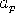and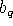for each origin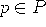and destination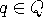so that when multiplied to the corresponding rows and columns of the prior matrix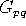the marginal totals of resulting matrix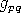correspond to the given productions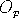and attractions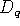. This can be expressed with the following set of equations: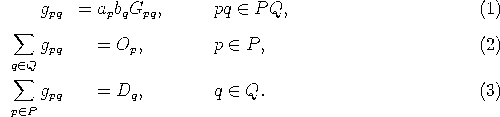It is easy to show that the above problem can also be expressed as the following convex optimization problem (also referred to as maximum entropy transportation problem):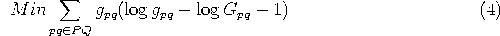subject to (2) and (3).

Defining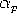and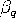as the dual variable associated with constraints (10) and (11), we obtain from the Kuhn-Tucker optimality conditions the following functional form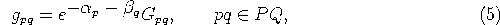which correspond exactly to (1) when using the following substitutions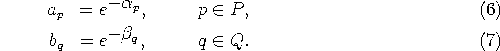The dual formulation of problem (4) can be written as the following unconstrained optimization problem: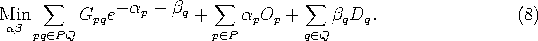The standard biproportional matrix balancing model is know to be always feasible when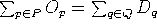and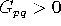for all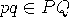.

Problems which have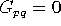for some O-D pairs can also be handled in this framework. But in this case, the O-D pairs withare not to be considered part of the set PQ and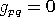is used for these O-D pairs where necessary, e.g. where it appears in a sum over origins or destinations.

Note, however, that problems withfor some O-D pairs are not always feasible. In this case, there is no easy method to determine the feasibility of a problem a priori, so that the primal feasibility of a solution found by a dual algorithm always has to be checked explicitly.

# Biproportional Matrix Balancing with Upper Bounds

We now turn our attention to an extension of the model defined in the previous section in which explicit upper bounds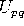are imposed on the resulting matrix elements. Since imposing upper bounds will have a direct impact on the functional form of the resulting model, it is not possible to formulate the extended model by simply adding a further constraint to problem (1)-(11).

However, the minimization problem (4) lends itself very well for an extension by adding upper bound constraints. This leads to the following extended problem formulation: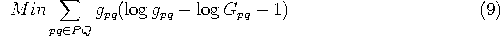subject to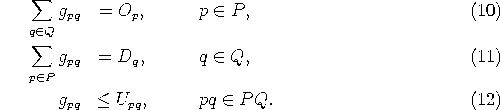Using againandas the dual variables associated with the production (=refprod) and attraction constraints (=refattr) and the new dual variables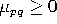for the upper bound constraints (12), we can state the Kuhn-Tucker optimality conditions for the above problem as follows: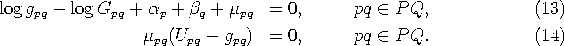The optimality condition (13) can be rewritten to yield the following functional form for the resulting model: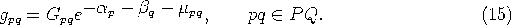By applying the complementary slackness conditions (14) for the dual variable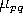and distinguishing between the cases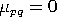and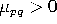, (15) becomes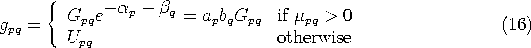which can be written even more concisely as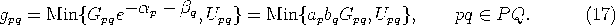Note that the above model formulation does no longer explicitly contain the dual variables.

Without loss of generality we can assume that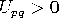for all, as matrix elementscan always be forced to zero by setting(and removing the corresponding O-D pairs from the set PQ, as explained in section 2).

The next step is to formulate the Lagrangian dual for problem (9) which becomes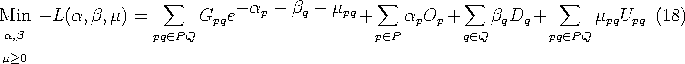Note that, except for the non-negativity of, the dual problem is essentially unconstrained. For this type of problem the a simple method of successive coordinate descent is know to converge to the optimal solution (see e.g. Luenberger 1984). Thus, it can be used to solve the dual problem (18) and find the optimal values of the dual variables. If the primal problem (9) is feasible, the optimal values of the dual variablesandcan be inserted into (17) to find the optimal solution of the primal problem.

Applying the coordinate descent method to the dual problem (18) means solving cyclically the first order optimality conditions for the corresponding dual variables. This implies finding the zeros for the partial derivatives of the dual objective function with respect to alland, and all non-zero: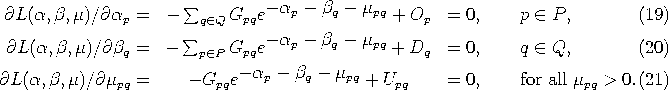By observing that (21) is equivalent to (17), it is easy to see that conditions (19) and (21) can be combined into the simpler production conditions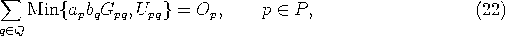and the conditions (20) and (21) combine into the simpler attraction conditions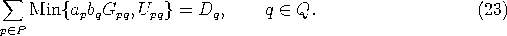Solving (22) forwill simultaneously satisfy the first order conditions (19) for origin p and (21) for allrelated to origin p. In the same manner solving (23) forwill simultaneously satisfy the first order conditions (20) for destination q and (21) for allrelated to destination q.

Before turning our attention to the solution algorithm, let us briefly look at the question of feasibility. The introduction of upper bounds influences the feasibility in a similarly complex manner as do the zeroes in the matrix. While of course the conditions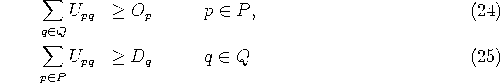are necessary conditions for feasibility, they are by no means sufficient.

# Solution Algorithm

The proposed solution algorithm can be classified as a coordinate descent method applied to the dual problem formulation. As it is based on iteratively solving equations (22) and (23), it actually does not optimize along dual coordinates one by one, but always satisfies the first order conditions simultaneously for all dual variables associated with either one origin or one destination, (which should result in an improved convergence compared to a one-by-one coordinate descent method).

Algorithm 1 describes the basic algorithm proposed to solve the biproportional matrix balancing problem with upper bounds (9). This algorithm assumes that equations of the type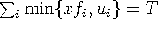can be solved for x. How this can be done efficiently is shown further on in Algorithm 2.

Algorithm 1:
0. Initialization

Set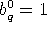forand set k:=1.
1. Balance origins

For each originsolve the equation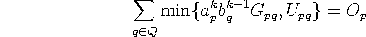for variable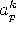by applying Algorithm 2.

2. Balance destinations

For each destinationsolve the equation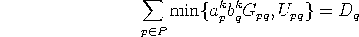for variable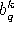by applying Algorithm 2.

3. Test stopping criteria

If convergence is reached for the the multipliersand,
e.g.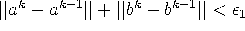, then go to step 4,

4. Compute primal solution

If the maximum production constraint violation for allis smaller than some predefined tolerance value, i.e.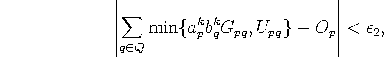then compute the optimal primal solution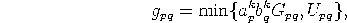otherwise the primal problem is infeasible. STOP.

Now let us consider the subproblem how to find the factor x which solves an equation of the form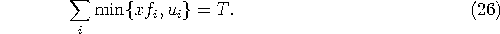This type of problem occurs in step 1 of Algorithm 1 with i=q,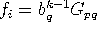,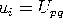, and in step 3 with i=q,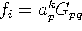,. Without loss of generality we can assume that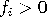and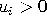for all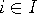. If there were any elements i with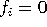and/or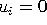, these can simply be dropped, since they do not influence the solution of (26) at all.

This type of problem can be solved by first sorting the elements i according to their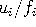ratios and then scanning the elements linearly in this order and checking if the optimal value x lies in the range between of two consecutiveratios. This leads to the formulation of the following algorithm:

Algorithm 2: (Solve)
0. Initialization

Set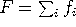and U=T
1. Sort elements

Sort the elements i in increasing order ofratios.
2. Scan elements

Using the order established in step 1, do for each i:
If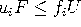then
set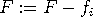and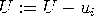,
else
go to step 3.
If this point is reached after having scanned all elements, then the problem is infeasible, STOP.
3. Compute optimal solution

Set the solution to x=U/F and STOP.

So far, the above algorithms have only been implemented in a ``quick and dirty'' manner purely for testing purposes. In the few examples tested so far (all based on data taken from the standard EMME/2 Winnipeg data bank using 154 traffic zones), the algorithm has been found to converge quite rapidly (4-7 iterations) to the optimal solution. But of course, further tests are needed.

# Conclusions

The methodology of introducing upper bounds is presented in this note only for the biproportional matrix balancing. However, it can most likely be applied directly to other related problems, such as the multi-proportional matrix balancing or Evans and Kirby's three-dimensional matrix balancing.

## References

1
Bregman L. (1967) The Relaxation Method of Finding the Common Point of Convex Sets and its Application to the Solution of Problems in Convex Programming. U.S.S.R. Computational Math. Mathematical Phys. 7, pp 200-217.

2
Evens S.P. and Kirby H.R. (1974) A Three-Dimensional Furness Procedure for Calibrating Gravity Models. Transportation Research, Vol 8, pp 105-122.

3
Furness K.P. (1970) Time Function Interaction. Traffic Engineering and Control Vol 7, No 7, pp19-36.

4
INRO Consultants Inc. (1996), EMME/2 User's Manual.

5
Lamond B. and Stewart N.F. (1981) Bregman's Balancing Method. Transportation Research, Vol 15B, pp 239-248.

6
Luenberger D.G. (1984) Linear and Nonlinear Programming. Second Edition, Addison-Wesley.

7
Murchland J. (1977) The Multi-proportional Problem. Univerity College London, research note JDM 263.

8
Spiess H. (1984). Contributions à la théorie et aux outils de planification de réseaux de transport urbain. Ph.D. thesis, Département d'informatique et de recherche opérationnelle, Centre de recherche sur les transports, Université de Montréal, Publication 382.
...
EMME/2 Support Center, Haldenstrasse 16, CH-2558 Aegerten, Switzerland

Heinz Spiess, EMME/2 Support Center, Wed Jun 4 13:03:46 MET DST 1997